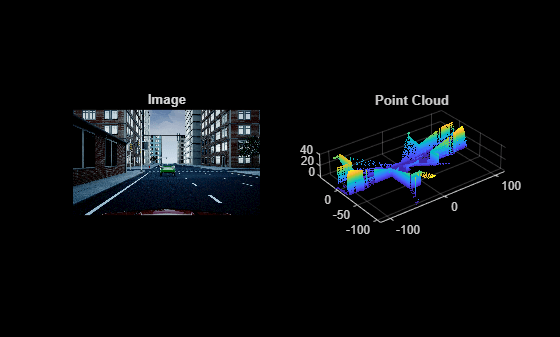# projectLidarPointsOnImage

Project lidar point cloud data onto image coordinate frame

Since R2020b

## Syntax

``imPts = projectLidarPointsOnImage(ptCloudIn,intrinsics,tform)``
``imPts = projectLidarPointsOnImage(worldPoints,intrinsics,tform)``
``[imPts,indices] = projectLidarPointsOnImage(___)``
``[___] = projectLidarPointsOnImage(___,Name,Value)``

## Description

example

````imPts = projectLidarPointsOnImage(ptCloudIn,intrinsics,tform)` projects lidar point cloud data onto an image coordinate frame using a rigid transformation between the lidar sensor and camera, `tform`, and a set of camera intrinsic parameters, `intrinsics`. The output `imPts` contains the 2-D coordinates of the projected points in the image frame.```
````imPts = projectLidarPointsOnImage(worldPoints,intrinsics,tform)` projects lidar points, specified as 3-D coordinates in the world frame, onto image coordinate frame.```
````[imPts,indices] = projectLidarPointsOnImage(___)` returns the linear indices of the projected points in the point cloud using any combination of input arguments in previous syntaxes.```
````[___] = projectLidarPointsOnImage(___,Name,Value)` specifies options using one or more name-value arguments in addition to any combination of arguments in previous syntaxes. For example, `'ImageSize',[250 400]` sets the size of the image on which to project the points to 250-by-400 pixels.```

## Examples

collapse all

Load ground truth data from a MAT-file into the workspace. Extract the image and point cloud data from the ground truth data.

```dataPath = fullfile(toolboxdir('lidar'),'lidardata','lcc','sampleColoredPtCloud.mat'); gt = load(dataPath); img = gt.im; pc = gt.ptCloud;```

Extract the camera intrinsic parameters from the ground truth data.

`intrinsics = gt.camParams;`

Extract the camera to lidar transformation matrix from the ground truth data, and invert to find the lidar to camera transformation matrix.

`tform = invert(gt.tform);`

Downsample the point cloud data.

`p1 = pcdownsample(pc,'gridAverage',0.5);`

Project the point cloud onto the image frame.

`imPts = projectLidarPointsOnImage(p1,intrinsics,tform);`

Overlay the projected points on the image.

```figure imshow(img) hold on plot(imPts(:,1),imPts(:,2),'.','Color','r') hold off```## Input Arguments

collapse all

Point cloud, specified as a `pointCloud` object.

Points in the world coordinate frame, specified as an M-by-3 matrix or M-by-N-by-3 array. If you specify an M-by-3 matrix, each row contains 3-D world coordinates of a point in an unorganized point cloud that contains M points in total. If you specify an M-by-N-by-3 array, M and N represent the number of rows and columns, respectively, in an organized point cloud. Each channel of the array contains the 3-D world coordinates of that point.

Data Types: `single` | `double`

Camera intrinsic parameters, specified as a `cameraIntrinsics` object.

Lidar to camera rigid transformation, specified as a `rigidtform3d` object.

### Name-Value Arguments

Specify optional pairs of arguments as `Name1=Value1,...,NameN=ValueN`, where `Name` is the argument name and `Value` is the corresponding value. Name-value arguments must appear after other arguments, but the order of the pairs does not matter.

Example: `ImageSize=[250 400]` sets the size of the image on which to project the points to 250-by-400 pixels.

Before R2021a, use commas to separate each name and value, and enclose `Name` in quotes.

Example: `'ImageSize',[250 400]` sets the size of the image on which to project the points to 250-by-400 pixels.

Indices selected for projection onto image coordinate frame, specified as a vector of positive integers.

Data Types: `single` | `double`

Size of the image on which the points are projected, specified as a two-element row vector of the form [width height] in pixels. The function uses the specified dimensions to filter out the projected points that are not in the field of view of the camera.

If you do no specify the `'ImageSize'` argument, then the function uses the `ImageSize` property from the camera intrinsic parameters `intrinsics` to estimate the field of view of the camera.

Note

If you specify an `'ImageSize'` argument greater than the default argument, then the function uses the default argument.

Data Types: `single` | `double`

## Output Arguments

collapse all

Points projected on image, returned as an M-by-2 matrix. Each row contains the 2-D coordinates, in the form [x y], a point in the image frame.

Data Types: `single` | `double`

Linear indices of the projected points of the point cloud, returned as a vector of positive integers.

Data Types: `single` | `double`

## Version History

Introduced in R2020b

expand all